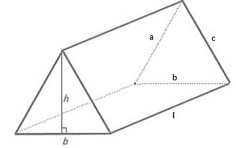# Surface Area of a Prism Formula

## Surface Area of a Prism Formula - Derivation & Solved Examples

A prism is a polyhedron that has two congruent parallel bases joined by parallelogram or rectangular (in the case of right prisms) faces laterally. The number of lateral surfaces is equal to the number of sides of the base polygon. For an n sided base polygon, the prism will have n rectangular faces. Therefore the total number of surfaces for a prism with an n sided polygonal base is $n + 2$. Consider such a prism whose length is h and whose side length and apothem of its base are respectively b and a. In general, the lateral surface area of a prism is equal to the product of the length of the prism and the perimeter of its base.
$LSA = {\text{perimeter}}\,{\text{of}}\,{\text{base}} \times h$
For a regular right prism, we have the following equations.
$\begin{gathered} TSA = 2 \times BA + LSA = 2 \times BA + n \times area\,of\,one\,rectangular\,face \hfill \\ \,\,\,\,\,\,\,\,\,\, = 2 \times \frac{n}{2} \times a \times b + n \times h \times b = nb\left( {a + h} \right) \hfill \\ \end{gathered}$
The following diagram shows the case of a triangular prism.$TSA = 2 \times BA + LSA = 2 \times \frac{1}{2} \times b \times h + l \times \left( {a + b + c} \right) = bh + l\left( {a + b + c} \right)$
In the case of a regular triangular prism, $a = b = c$. Therefore, we have the following formula for its surface area.
$TSA = bh + 3lb = b\left( {h + 3l} \right)$
Let’s look at an example to see how to apply these formulae.

Question: The two bases of a triangular prism are equilateral triangles of side length 4 cm. If its length is 6 cm, then find its total surface area.
Solution:
$n = 3,\,\,b = 4\,cm,\,\,h = 6\,cm$
$BA = \frac{{\sqrt 3 }}{4}{b^2} = \frac{{\sqrt 3 }}{4}{4^2} = 4\sqrt 3 \,c{m^2}$
$\begin{gathered} TSA = 2 \times BA + n \times area\,of\,one\,rectangular\,face \hfill \\ \,\,\,\,\,\,\,\,\,\, = 2 \times 4\sqrt 3 + 3 \times 4 \times 6 = 72 + 8\sqrt 3 = 8\left( {9 + \sqrt 3 } \right)\,c{m^2} \hfill \\ \end{gathered}$
Why don’t you try using these formulae to solve the following problem?

Question: A regular hexagonal prism is 12 cm long and the perimeter of its hexagonal base is 30 cm. Find its total surface area.
Options:
(a) $15\left( {12 + 15\sqrt 3 } \right)\,c{m^2}$
(b) $15\left( {24 + 5\sqrt 3 } \right)\,c{m^2}$
(c) $12\left( {25 + 5\sqrt 3 } \right)\,c{m^2}$
(d) $10\left( {24 + 5\sqrt 3 } \right)\,c{m^2}$
$n = 6,\,\,h = 12\,cm,\,\,perimeter\,of\,base = 30\,cm$
${\text{Perimeter}}\,{\text{of}}\,{\text{base}} = n \times b \Rightarrow 6 \times b = 30 \Rightarrow b = 5\,cm$
$BA = \frac{{3\sqrt 3 }}{2}{b^2} = \frac{{3\sqrt 3 \times {5^2}}}{2} = \frac{{75\sqrt 3 }}{2}\,c{m^2}$
$LSA = {\text{perimeter}}\,{\text{of}}\,{\text{base}} \times h = 30 \times 12 = 360\,c{m^2}$
$TSA = 2 \times BA + LSA = 2 \times \frac{{75\sqrt 3 }}{2} + 360 = 360 + 75\sqrt 3 = 15\left( {24 + 5\sqrt 3 } \right)\,c{m^2}$